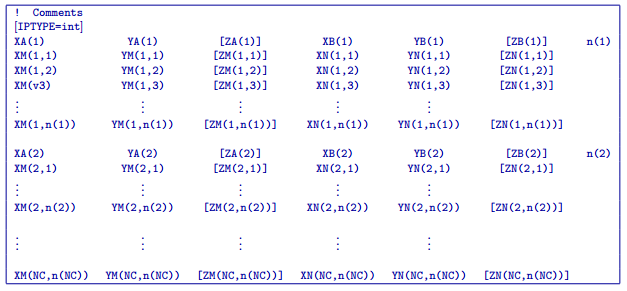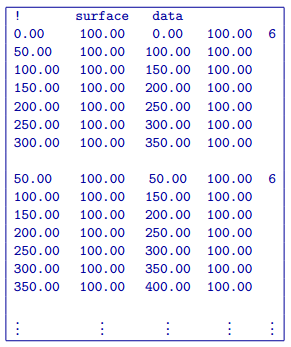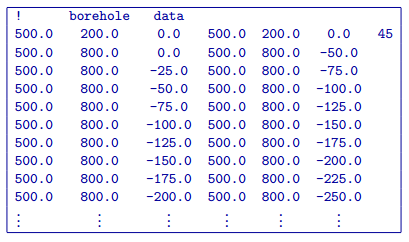# 5.1.8. DCIP3D locations files¶

## 5.1.8.1. Locations file¶

This file is used to specify current and potential electrode locations required for the forward modelling of DC/IP data. The locations file has the following structure:Parameter definitions:

!

Lines starting with ! are comments.

IPTYPE

A special directive that indicates the IP data type. This directive is only required in IP data files. The IPTYPE enables the IP inversion programs to distinguish the apparent chargeability and other similar IP measurements from the basic secondary potentials.

• IPTYPE = 1 is commonly used for IP data in which apparent chargeability is well defined (i.e. using dimensionless apparent chargeability, integrated chargeability, PFE, or phase data acquired using electrode configurations that do not produce zero crossings in the measured total potential). The following are some examples of this type of geometry: any pole-pole array (surface or borehole), surface pole-dipole or dipole-dipole array along the same traverse, gradient arrays where the potential electrodes are parallel to the current electrodes, or borehole pole-dipole or dipole-dipole array with all active electrodes in the same borehole.

• IPTYPE = 2 is used for secondary potential IP data measured using any electrode geometry. This is typically used when cross-line surface data or cross-hole borehole data are inverted. For these array geometries, the apparent chargeability cannot be defined since the total potential can be zero.

• The dimensionless apparent chargeabilities (IPTYPE = 1) and the secondary potentials (IPTYPE = 2) can be mixed in the same file. Thus an IP data file can have several occurrences of IPTYPE. All the data are treated as the same type following an IPTYPE directive until a new line changes the type.

$$XA(i),YA(i),ZA(i)$$

Location (X,Y,Z) of the $$i^{th}$$, current electrode A (measured in metres).

$$XB(i),YB(i),ZB(i)$$

Location (X,Y,Z) of the $$i^{th}$$, current electrode B (measured in metres).

$$XM(i,j),YM(i,j),ZM(i,j)$$

Location (X,Y,Z) of the $$j^{th}$$ potential electrode M, corresponding with the $$i^{th}$$ current electrode or electrode pair (measured in metres).

$$XN(i,j),YN(i,j),ZN(i,j)$$

Location of the $$j^{th}$$, potential electrode N corresponding with the $$i^{th}$$ current electrode or electrode pair (measured in metres).

$$NC$$

The total number of current electrodes or electrode pairs.

NOTE: The brackets $$[\cdots]$$ indicate that the enclosed parameter is optional. The Z location of the electrodes is optional if you are working only with surface data (i.e. your electrodes are draped to topography) and the IPTYPE only needs to be specified if you are working with IP data.

## 5.1.8.2. Examples of a locations file¶

We provide two example files below. The first file is for a simple surface dataset while the second file shows how borehole data can be incorporated.

Example of surface data locations:Example with borehole data locations: